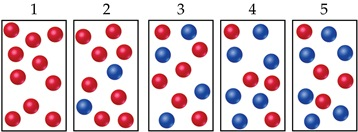# Problem: The following diagrams represent a hypothetical reaction A , with A represented by red spheres and B represented by blue spheres. The sequence from left to right represents the system as time passes.Does the system reach equilibrium?

###### FREE Expert Solution

We are asked if the system reached equilibrium.

A reaction reaches equilibrium once the rate of the forward reaction equals the rate of the reverse reaction.

Once at equilibrium there is no net change in the concentration of reactants or products.

79% (196 ratings)###### Problem Details

The following diagrams represent a hypothetical reaction A , with A represented by red spheres and B represented by blue spheres. The sequence from left to right represents the system as time passes.Does the system reach equilibrium?